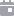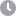Read time: 5 minutes

# Tracer Perpetual Pools: Volatility Decay

The impact of Volatility Decay on Tracer Perpetual Pools## Overview

Tracer's new financial contract, Perpetual Pools, is most similar to a decentralised Leveraged ETF (LETF). If you are familiar with LETFs, then you would know they suffer from a phenomenon named Volatility Decay. Perpetual Pools v1 is no different. Volatility decay, put simply, is the decay in a traders PnL (profit and loss) due to frequent directional change in the price of the underlying asset. While Perpetual Pools suffer from this, simulations suggest that their returns more closely track naive expectations of leverage than LETFs. That being said, we suggest traders use these instruments only for short term directional bets rather than hold them.

## Introduction

The Tracer: Perpetual Pools Litepaper outlines a simple derivative design to create leveraged tokens, in which long and short users have changing claims on a pool of collateral. In this design, the proportion of collateral that each party can claim changes based on a leveraged function of the underlying asset. This means that shares of the pool, which are tokenised, are leveraged. While a two-sided pool is the simplest way to gain leveraged exposure, and can't be liquidated like a perpetual swap, it comes with a downside – volatility decay.

## What is Volatility Decay?

Volatility decay, put simply, is the decay in a traders PnL (profit and loss) due to frequent directional change in the price of the underlying asset. It's the difference between the naive expectation for leveraged returns and the actual return of rebalanced leverage products like LETFs, leveraged tokens (FTX, Binance, ETH2x-FLI) and Perpetual Pools. The instruments mentioned are designed for short term directional bets as the returns are path-dependent. They should not be held long term. This article will break down why volatility decay occurs and how it relates to Perpetual Pools v1.

The following graph shows the decay of a Perpetual Pool long trader's PnL at a power leverage of 2, as the trader experiences sideways volatility. If this graph makes sense to you, then that's all you need to know. If it doesn't, read on below.## Effective Leverage

LETFs are a simple example of a rebalanced leverage product. These funds track an index that targets a specific leverage at the end of each trading day. You'll get a better grasp on this if you can understand the distinction between leverage and effective leverage. Let's compare LETFs and perpetual swaps to make this distinction. The chart below shows the effective leverage of a 2x perpetual swap long positionThe effective leverage on a perpetual swap varies with the price of the underlying. As the price goes up, the value of your long position increases. When this happens, your position's profit pays back some of the amount you borrowed to open the position. Because the leverage multiplier is based on your initial opening price, as the value of the portfolio grows, your leverage shrinks. If you wanted to maintain a certain leverage multiplier with a perpetual swap, it would require constant rebalancing. That means adding and removing margin to maintain your debt to equity ratio. This is where Perpetual Pools and LETFs are advantageous.LETFs rebalance at the end of each trading day. They rebalance to target the same leverage by reinvesting profits back into the position as margin. If the position loses money during the day, they will decrease their overall debt. The effective leverage curve of an LETF is, thus, constant: it's the flat line in the image above. Take an example where the price goes up on consecutive days. An LETF will profit more than a perpetual swap as daily profits are reinvested back into the position (a side note: profits are reinvested as margin to support more borrowings). This is the act of rebalancing. But rebalancing also means that the position stands to lose more when price goes down. Consider the example where price goes from 100→101→100→101:Image 4: In this table, the pnl columns represent cumulative PnL for both perpetual Swaps and LETFs.

There's a difference between the LETF's return and the perpetual swap's return. This difference is what we call volatility decay: the price moved sideways (it was volatile) and, though the underlying returned to the same starting price, the act of rebalancing manifests as a decay in the performance of the LETF. At time 3, the perpetual swap's returns are 0.02% greater than that of the LETF. LETFs, and similar rebalancing assets, are made for short term, unidirectional trades as there will be decay every time there's a price reversion.

## Perpetual Pools Performance

We can also compare the volatility decay of LETFs to Perpetual Pools v1. First, assume that LETF performance follows from this equation derived in Zhang 2010:This equation looks complicated, but it's actually just accounting for management tracking error and expense ratios of the LETF (Zhang 2010). Perpetual Pools don't have tracking error or high expense ratios that eat into a traders PnL.

Under two months of historical ETH/USD data (fig. 1), LETFs perform worse than Perpetual Pool leveraged tokens. LETF performance (orange) was generated using Zhang's equation (2.2.4). In fairness, the best case scenario is assumed, where the expense ratio r, funding rate f and cost of borrowing $\lambda$ is set to 0. The performance of a Perpetual Pool leveraged long token is generated alongside (blue), using the same price data.Over the 2 months, when ETH started and ended on roughly the same price, both assets suffer from volatility decay. However, Perpetual Pool leveraged tokens suffer less when compared to an hourly rebalanced LETF.

## Pathway Forward

Perpetual Pools v1 is an effective instrument for short-term directional bets.

The aim of Perpetual Pools v2 is to supports long-term directional bets. If the transfer function can address or eliminate structural volatility decay, v2 leveraged tokens will be suitable for long-term holders. Mycelium and Paperclip Fund are actively researching a solution. More details to come in future publications.Tracer Perpetual Pools V1 is currently live on Arbitrum One. Fully fungible, leveraged tokens for the DeFi economy, with no margin requirements and no liquidations.Read more

Launch Perpetual Pools